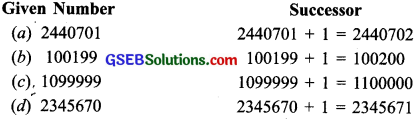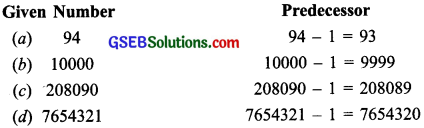# GSEB Solutions Class 6 Maths Chapter 2 Whole Numbers Ex 2.1

Gujarat Board GSEB Textbook Solutions Class 6 Maths Chapter 2 Whole Numbers Ex 2.1 Textbook Questions and Answers.

## Gujarat Board Textbook Solutions Class 6 Maths Chapter 2 Whole Numbers Ex 2.1

Exercise 21 (Page 31)

Question 1.
Write (he next three natural numbers after 10999.
Solution:
The next three natural numbers after 10999
are: 11000. 11001 and 11.002.

Question 2.
Write the three whole numbers occurring just before 10001.
Solution:
The three whole numbers occurring just before 10001 are: 10000, 9999 and 9998.

Question 3.
Which is the smallest whole number?
Solution:
The smallest whole number is 0.

Question 4.
How many whole numbers are there between 32 and 53?
Solution:
The whole numbers between 32 and 53 are:
33, 34, 35, 36, 37, 38, 39, 40, 41, 42, 43, 44,
45, 46, 47, 48, 49, 50, 51, 52 and there are 20 whole numbers.
There are 20 whole numbers between 32 and 53.Question 5.
Write the successor of
(a) 2440701
(b) 100199
(c) 1099999
(d) 2345670
Solution:Question 6.
Write the predecessor of
(a) 94
(b) 10000
(c) 208090
(d) 7654321
Solution:Question 7.
In each of the following pairs of numbers, state which whole number is on the left of the other number on the number line. Also write them with the appropriate sign (>, <) between them. (a) 530, 503 (b) 370, 307 (c) 98765, 56789 (d) 9830415, 10023001 Solution: (a) 530, 503 On the number line, the whole number 503 is on the left of 530. 530 > 503

(b) 370, 307
On the number line, the whole number
307 is on the left of 370.
370 > 307

(c) 98765, 56789
On the number line, the whole number
56789 is on the left of 98765.
98765 > 56789

(d) 9830415, 10023001:
On the number line, the whole number
9830415 is on the le’ft of 10023001.
10023001 > 9830415Question 8.
Which of the following statements are true
(T) and which are false (F)?
(a) Zero is the smallest natural number
(b) 400 is the predecessor of 399.
(c) Zero is the smallest whole number
(d) 600 is the successor of 599.
(e) All natural numbers are whole numbers.
(f) All whole numbers are natural numbers.
(g) The predecessor of a two-digit number is never a single-digit number
(h) I is the smallest whole number
(i) The natural number I has no predecessor
(j) The whole number 1 has no predecessor
(k) The whole number 13 lies between 11 and 12.
(l) The whole number O has no predecessor
(m)The successor of a two-digit number is always a two-digit number
Solution:
(a) False statement (F)
(b) False statement (F)
(c) True statement (T)
(d) True statement (T)
(e) True statement (T)
(f) False statement (F)
(g) False statement (F)
(h) False statement (F)
(i) True statement (T)
(J) False statement (F)
(k) False statement (F)
(l) True statement (T)
(m)False statement (F)